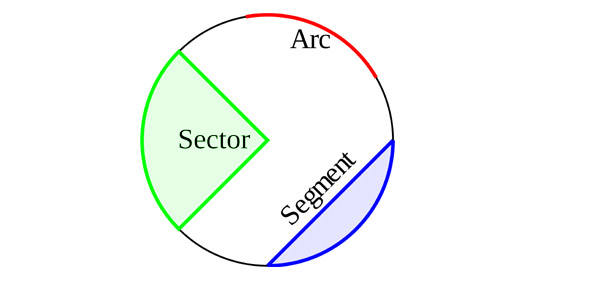5 Questions | Total Attempts: 427SettingsYou must be able to change the diameter to a radius in order to find the area of a circle. Practice that skill now. Remember that inorder to change a radius to a diameter you MULTIPLY by 2. To change a diameter to a radius you DIVIDE by 2.

Related Topics
• 1.
A circle has a radius of 10 cm, what is the diameter of the circle?
• A.

5 cm

• B.

20 cm

• C.

10 cm

• D.

12 cm

• 2.
A circle has a diameter of 30 in, what is the radius of this circle?
• A.

15 in

• B.

60 in

• C.

10 in

• 3.
Max has a plate with a diameter of 25 inches.  For Max to find the area of the plate he has to know the radius, what is the radius of the plate?
• A.

12 in

• B.

50 in

• C.

12.5 in

• 4.
Sue has a bicycle wheel with a radius of 6.5 in.  What is the diameter of Sue's wheel?
• A.

65 in

• B.

13 in

• C.

3.25 in

• D.

3 in

• 5.
Payton has a yo-yo with a diameter of 4 cm, what is the radius of the yo-yo?
• A.

4 cm

• B.

8 cm

• C.

2 cm